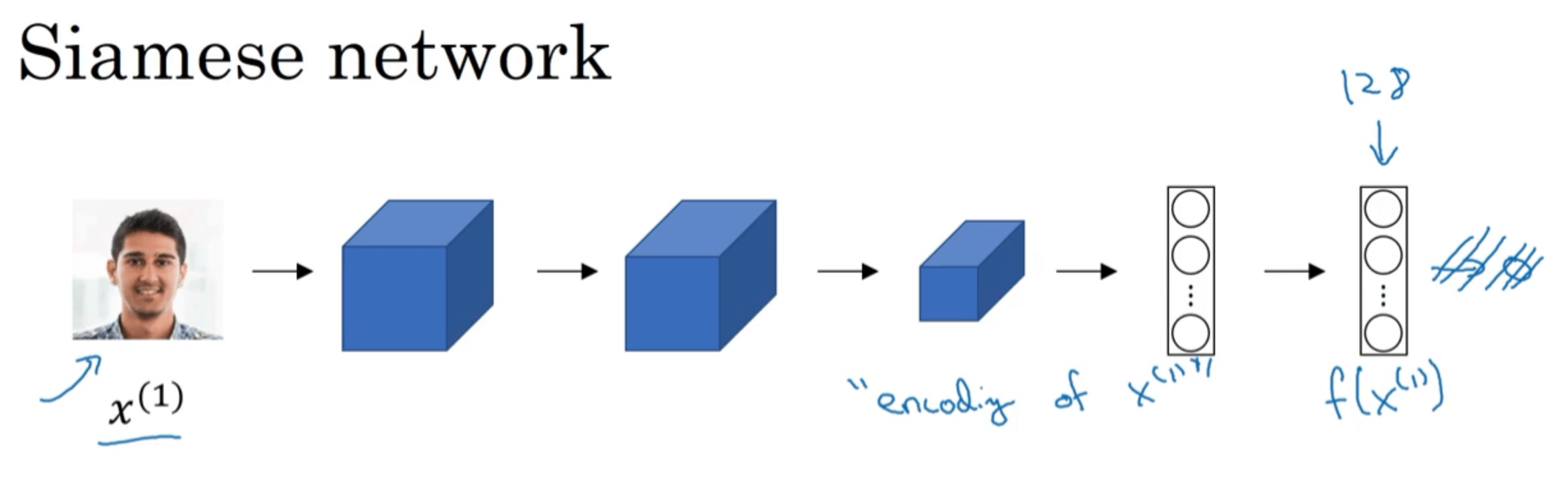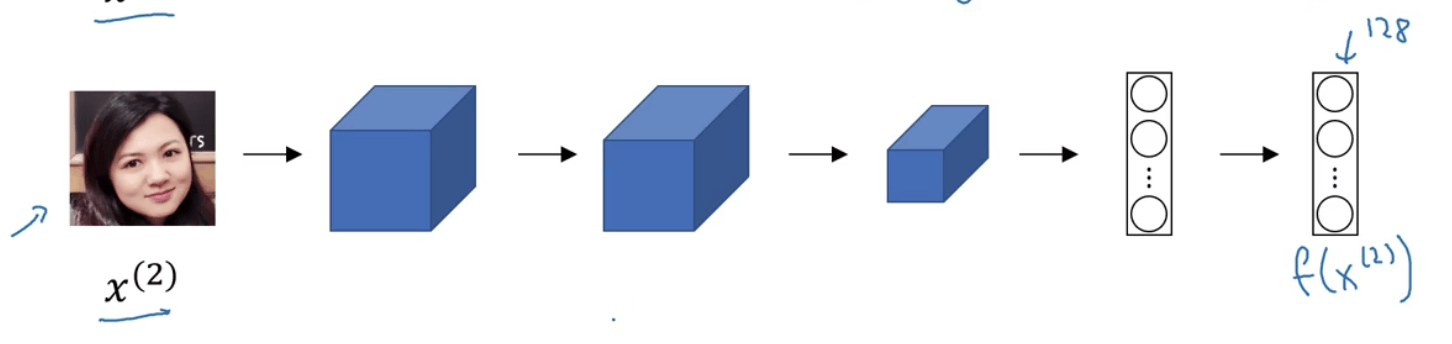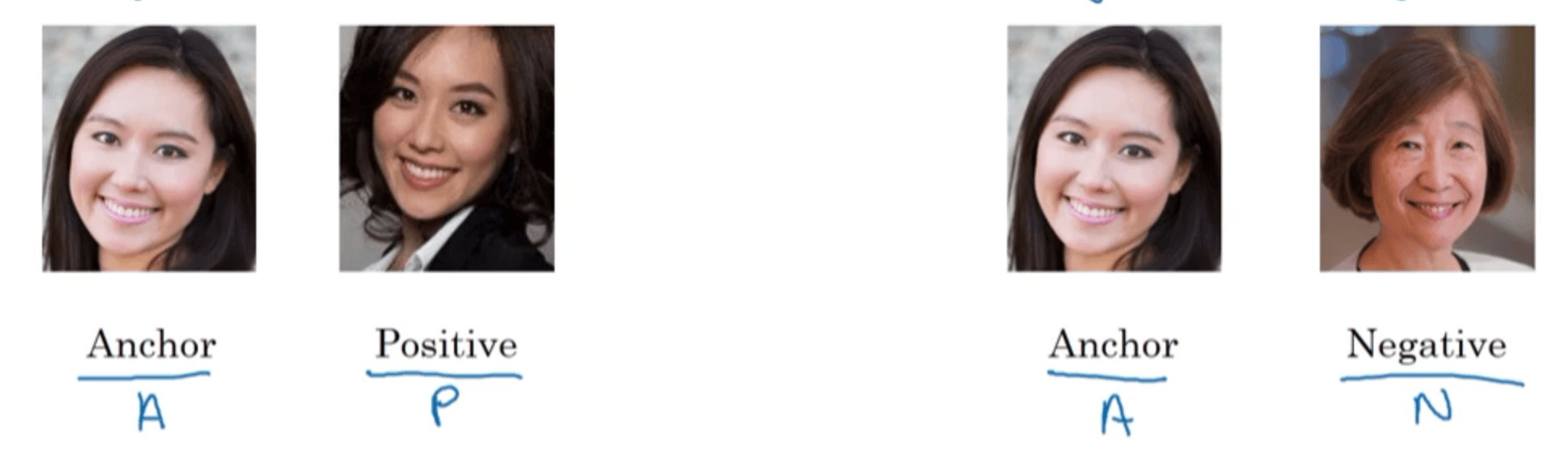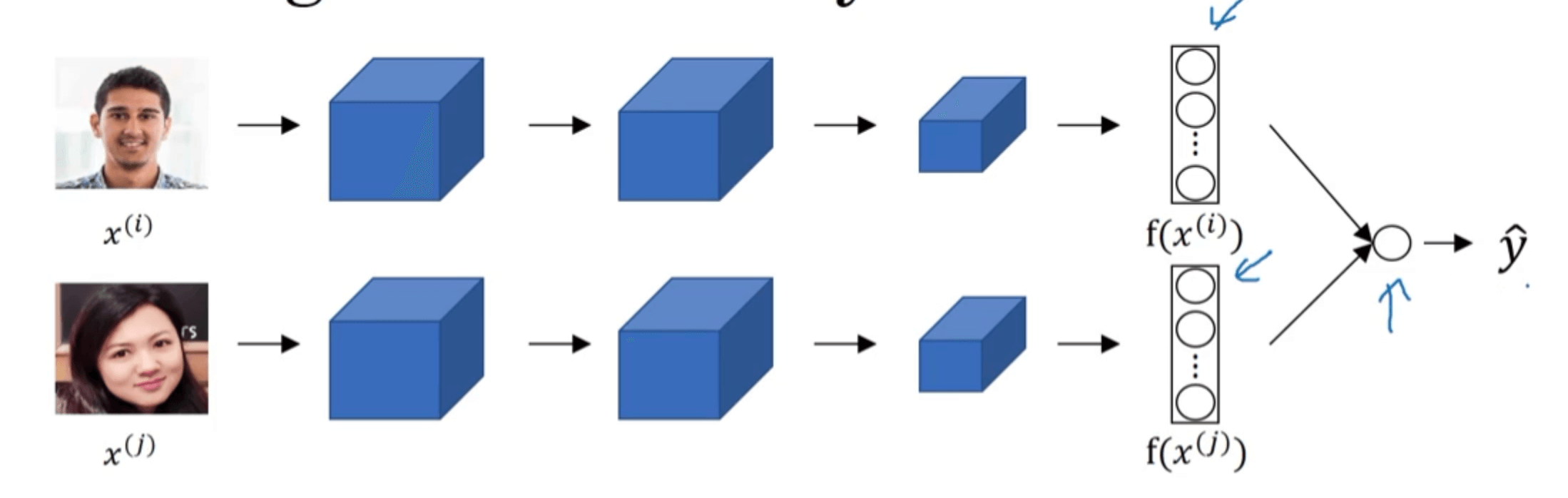# 【深度学习基础】第三十六课：人脸识别

## One-Shot Learning，Siamese网络，Triplet Loss Function

Posted by x-jeff on September 24, 2020

【深度学习基础】系列博客为学习Coursera上吴恩达深度学习课程所做的课程笔记。

# 2.Siamese网络

Siamese译为“连体，孪生”之意，因此Siamese网络指的是两个或多个一模一样的网络。$d(x^{(1)},x^{(2)})=\lVert f(x^{(1)})-f(x^{(2)}) \rVert _2 ^2$

# 3.Triplet Loss Function• A : anchor image
• P : positive image（和A属于同一个人）
• N : negative image（和A不是同一个人）

$d(A,P) \leqslant d(A,N)$

$d(A,P) - d(A,N) \leqslant 0$

$d(A,P) - d(A,N) + \alpha \leqslant 0 \tag{1}$

$\alpha$被称作“间隔”，是一个超参数（$\alpha > 0$）。

$L(A,P,N)=max(d(A,P) - d(A,N) + \alpha,0)$

$J=\sum_{i=1}^m L(A^{(i)},P^{(i)},N^{(i)})$

# 4.人脸识别与二分类

Triplet Loss Function是学习Siamese网络参数的一种办法，本节将介绍其他学习Siamese网络参数的方法：将人脸识别转换成一个二分类问题。$\hat{y}=logistic(\sum_{k=1}^{128} w_k \left\vert f(x^{(i)})_k - f(x^{(j)})_k \right\vert +b)$

$\hat{y}=logistic(\sum_{k=1}^{128} w_k \frac{(f(x^{(i)})_k - f(x^{(j)})_k)^2}{ f(x^{(i)})_k + f(x^{(j)})_k}+b)$

$\hat{y}=logistic(\sum_{k=1}^{128} w_k \chi ^2+b)$

Ps：为了提升识别效率，可以将训练好的模型为数据库中每一位员工生成其特定的特征向量并保存。当有访客来临时，只需计算访客的特征向量，并与已保存好的员工特征向量进行比较即可。

# 5.代码地址